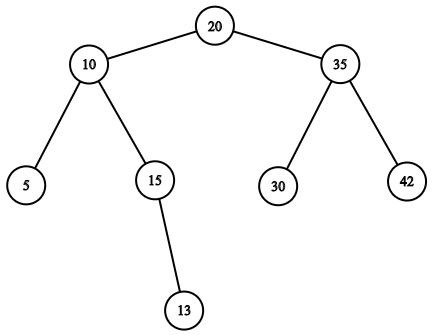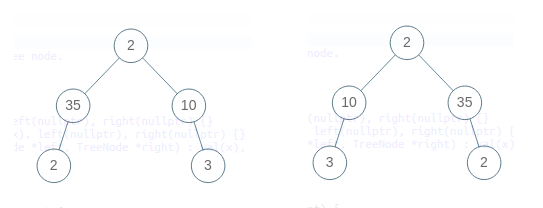1

# Isomorphic Trees

Difficulty: EASY
Avg. time to solve
32 min
Success Rate
67%

Problem Statement
Suggest Edit

#### Two binary trees are said to be isomorphic with each other, if one of the trees can be obtained from the other, by performing the following operation any number of times:

``````Choose a node of the tree, swap its left and right subtree i.e the left subtree will become the right one, and vice versa.
``````
##### Note:
``````1. A binary tree is a tree in which each node has at most two children.

2. The left subtree of a node, is the tree in which the left child of the node is the root of that tree, and the same holds for the right subtree.

3. The given operation can be performed on any node at any level of the given trees.

4. Two empty trees are also said to be isomorphic.
``````
##### Input Format:
``````The first line contains a single integer t representing the number of test cases.

The first line of each test case will contain the values of the nodes of the first tree in the level order form ( -1 for NULL node).

The second line of each test case will contain the values of the nodes of the second tree in the level order form ( -1 for NULL node). Refer to the example for further clarification.

Example:
Consider the binary tree:
````````````The input of the tree depicted in the image above will be like:

20 10 35 5 15 30 42 -1 -1 -1 13 -1 -1 -1 -1 -1 -1
``````
##### Explanation:
``````Level 1 :
The root node of the tree is 20

Level 2 :
Left child of 20 = 10
Right child of 20 = 35

Level 3 :
Left child of 10 = 5
Right child of 10 = 15
Left child of 35 = 30
Right child of 35 = 42

Level 4 :
Left child of 5 = null (-1)
Right child of 5 = null (-1)
Left child of 15 = null (-1)
Right child of 15 = 13
Left child of 30 = null (-1)
Right child of 30 = null (-1)
Left child of 42 = null (-1)
Right child of 42 = null (-1)

Level 5 :
Left child of 13 = null (-1)
Right child of 13 = null (-1)

The first not-null node (of the previous level) is treated as the parent of the first two nodes of the current level. The second not-null node (of the previous level) is treated as the parent node for the next two nodes of the current level and so on.

The input ends when all nodes at the last level are null (-1).
``````
##### Output Format:
``````For each test case, return “yes” if both trees are isomorphic, “no” otherwise.
``````
##### Note:
``````You do not need to print anything, it has already been taken care of. Just implement the given function.
``````
##### Constraints:
``````1 <= T <= 100
0 <= N <= 10^3
1 <= nodeValue <= 10^6

Time Limit: 1sec
``````
##### Sample Input 1:
``````1
2 35 10 2 -1 -1 3 -1 -1 -1 -1
2 10 35 3 -1 -1 2 -1 -1 -1 -1
``````
##### Sample Output 1:
``````yes
``````
##### Explanation of the Sample Input1:``````The first tree can be converted into the second one using the swap operation three times as follows:
Swap the left and right subtree of the node with the value = 2.
Swap the left and right subtree of the node with the value = 35.
Swap the left and right subtree of the node with the value = 10.
``````
##### Sample Input 2:
``````1
2 35 10 2 3 -1 -1 -1 -1 -1 -1
9 10 35 -1 -1 3 2 -1 -1 -1 -1
``````
##### Sample Output 2:
``````no
``````Console